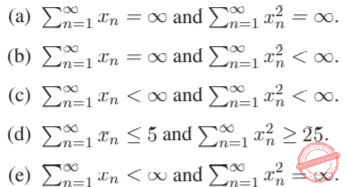## TIFR PHD CS & SS 2014

 Question 1
Consider the reactions
X + 2Y → 3Z
2X + Z → Y.
Let nX, nY , nZ denote the numbers of molecules of chemicals X, Y , Z in the reaction chamber. Then
which of the following is conserved by both reactions?
 A nX + nY + nZ B nX + 7nY + 5nZ C 2nX + 9nY - 3nZ D 3nX - 3nY + 13nZ E None of the above
 Question 2
A body at a temperature of 30 Celsius is immersed into a heat bath at 0 Celsius at time t = 0. The body starts cooling at a rate proportional to the temperature difference. Assuming that the heat bath does not change in temperature throughout the process, calculate the ratio of the time taken for the body to reach 1 Celsius divided by the time taken for the body to reach 5 Celsius.
 A log 5 B log 29/log 25 C e5 D 1 + log6 5 E None of the above
 Question 3
The Fibonacci sequence is defined as follows: F0 = 0, F1 = 1, and for all integers n ≥ 2,
Fn = Fn-1 + Fn-2. Then which of the following statements is FALSE?A a B b C c D d E e
Engineering-Mathematics       Combinatorics
 Question 4
Consider numbers greater than one that satisfy the following properties:
(a) they have no repeated prime factors;
(b) for all primes p ≥ 2, p divides the number if and only if p − 1 divides the number.
The number of such numbers is
 A 0 B 5 C 100 D Infinite E None of the above
Engineering-Mathematics       Combinatorics
 Question 5
The rules for the University of Bombay five-a-side cricket competition specify that the members of each team must have birthdays in the same month. What is the minimum number of mathematics students needed to be enrolled in the department to guarantee that they can raise a team of students?
 A 23 B 91 C 60 D 49 E None of the above
Engineering-Mathematics       Combinatorics
 Question 6
Karan tells truth with probability 1/3 and lies with probability 2/3. Independently, Arjun tells truth with probability 3/4 and lies with probability 1/4. Both watch a cricket match. Arjun tells you that India won, Karan tells you that India lost. What probability will you assign to India’s win?
 A 1/2 B 2/3 C 3/4 D 5/6 E 6/7
Engineering-Mathematics       Probability-and-statistics
 Question 7
Consider a sequence of non-negative numbers {xn : n = 1, 2,...}. Which of the following statements cannot be true?A a B b C c D d E e
Engineering-Mathematics       Combinatorics
 Question 8
All that glitters is gold. No gold is silver.
Claims:
1. No silver glitters.
2. Some gold glitters.
Then, which of the following is TRUE?
 A Only claim 1 follows B Only claim 2 follows C Either claim 1 or claim 2 follows but not both D Neither claim 1 nor claim 2 follows E Both claim 1 and claim 2 follow
Aptitude       Numerical
 Question 9
Solve min x2 + y2
subject to
x + y ≥ 10,
2x + 3y ≥ 20,
x ≥ 4,
y ≥ 4.
 A 32 B 50 C 52 D 100 E None of the above
Engineering-Mathematics       Inequality
 Question 10
A person went out between 4pm and 5pm to chat with her friend and returned between 5pm and 6pm. On her return, she found that the hour-hand and the minute-hand of her (well-functioning) clock had just exchanged their positions with respect to their earlier positions at the time of her leaving. The person must have gone out to chat at
 A Twenty five minutes past 4pm B Twenty six and 122/143 minutes past 4pm C Twenty seven and 1/3 minutes past 4pm. D Twenty eight minutes past 4pm E None of the above
Aptitude       Numerical
 Question 11
A large community practices birth control in the following peculiar fashion. Each set of parents continues having children until a son is born; then they stop. What is the ratio of boys to girls in the community if, in the absence of birth control, 51% of the babies are born male?
 A 51:49 B 1:1 C 49:51 D 51:98 E 98:51
Aptitude       Numerical
 Question 12A The above inequality holds under conditions (1) and (2) but not under condition (3). B The above inequality holds under conditions (2) and (3) but not under condition (1) C The above inequality holds under conditions (1) and (3) but not under condition (2). D The above inequality holds under all the three conditions E The above inequality holds under none of the three conditions
Engineering-Mathematics       Inequality
 Question 13
Let L be a line on the two dimensional plane. L’s intercepts with the X and Y axes are respectively a and b. After rotating the co-ordinate system (and leaving L untouched), the new intercepts are a and b respectively. Which of the following is TRUE?A a B b C c D d E e
Engineering-Mathematics       Co-ordinate-Geometry
 Question 14
Let m and n be any two positive integers. Then, which of the following is FALSE?
 A m + 1 divides m2n − 1 B For any prime p, mp ≡ m (modp) C If one of m, n is prime, then there are integers x, y such that mx + ny = 1 D If m E If 2n − 1 is prime, then n is prime.
Aptitude       Numerical
 Question 15A a B b C c D d E e
Engineering-Mathematics       Calculus
There are 15 questions to complete.

Register Now